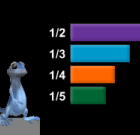## Thursday, October 4, 2012

### Problem Solving with FractionsThis fraction game introduces students to a character with an interesting dilemma. Walker wants desperately to get home but the road has gaps that prevent him from reaching his destination. At each gap, Walker is presented with increasingly challenging tasks involving fraction pieces. Students must help Walker solve these problems in order to move him closer to home. In the process, students apply their knowledge of fractions to an engaging problem within a meaningful context. Students work primarily with unit fractions at first but are later shown how to build any fraction needed.

Fraction concepts covered in this game include:
• unit fractions
• equivalent fractions
• comparing fractions
• adding and subtracting fractions
• multiplying and dividing fractions

This game is best presented as a whole group activity before students explore on their own. An interesting component of the game is a tool called the combinator. After solving simple challenges with unit fractions, students are introduced to higher level fractions such as 2/3 and 3/4. Students build these fractions by physically dragging unit fractions to the combinator. This tool provides a powerful visual for students who are beginning to develop a deeper understanding of fractions and how they are composed.